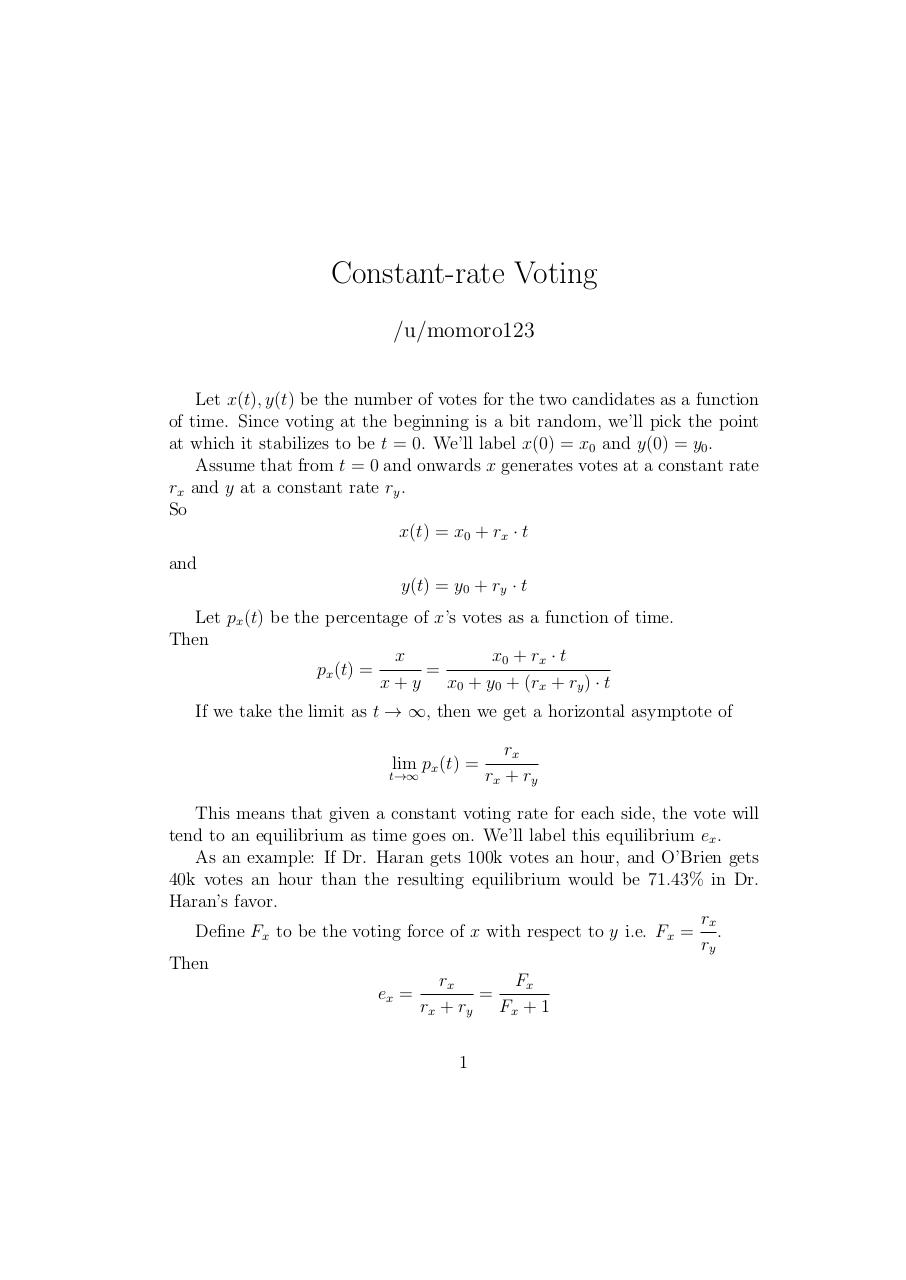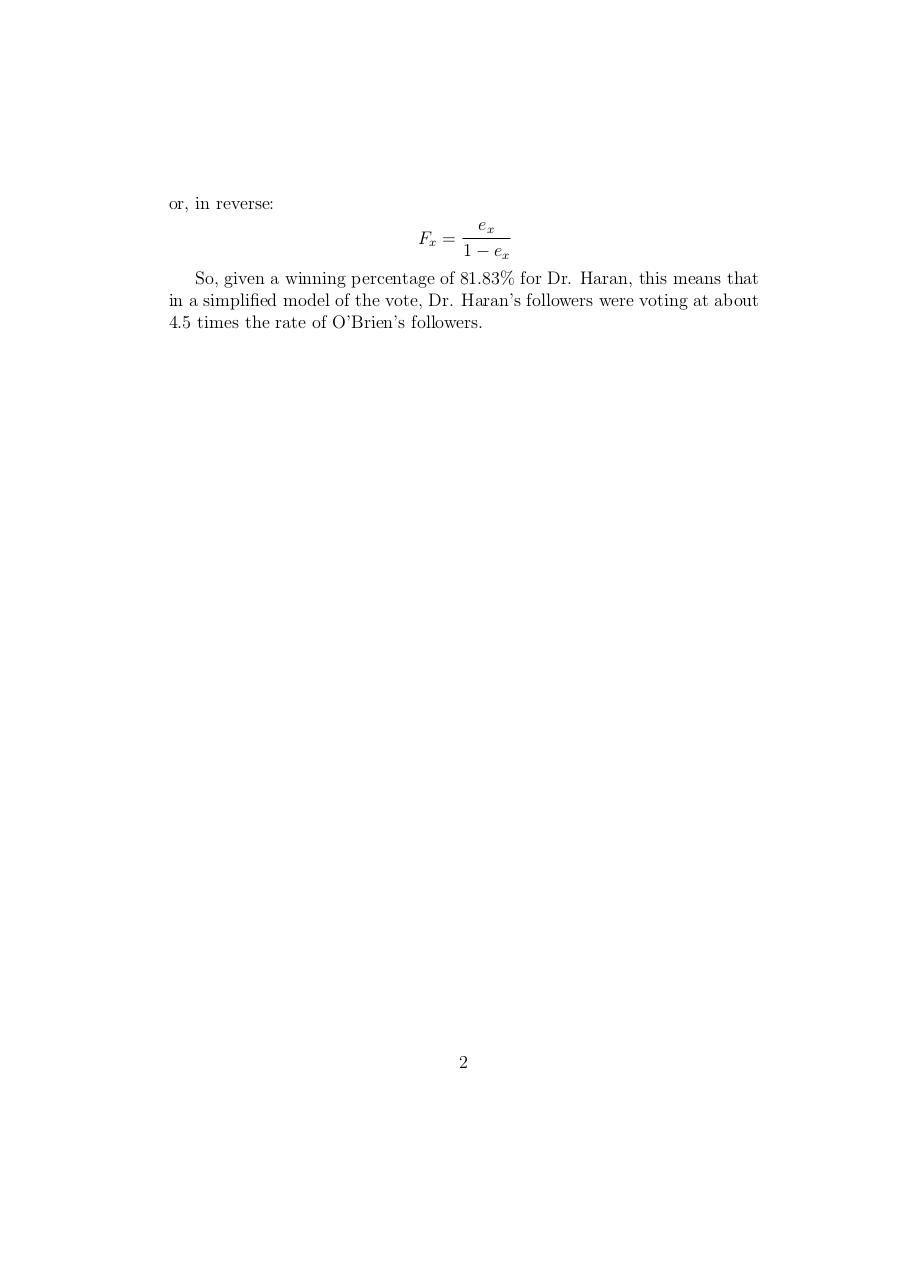# Constant rate Voting .pdf

### File information

Original filename: Constant_rate_Voting.pdf

This PDF 1.5 document has been generated by TeX / pdfTeX-1.40.18, and has been sent on pdf-archive.com on 26/07/2017 at 11:55, from IP address 212.179.x.x. The current document download page has been viewed 641 times.
File size: 69 KB (2 pages).
Privacy: public file

Constant_rate_Voting.pdf (PDF, 69 KB)

### Document preview

Constant-rate Voting
/u/momoro123
Let x(t), y(t) be the number of votes for the two candidates as a function
of time. Since voting at the beginning is a bit random, we’ll pick the point
at which it stabilizes to be t = 0. We’ll label x(0) = x0 and y(0) = y0 .
Assume that from t = 0 and onwards x generates votes at a constant rate
rx and y at a constant rate ry .
So
x(t) = x0 + rx · t
and
y(t) = y0 + ry · t
Let px (t) be the percentage of x’s votes as a function of time.
Then
x0 + r x · t
x
=
px (t) =
x + y x0 + y0 + (rx + ry ) · t
If we take the limit as t → ∞, then we get a horizontal asymptote of
lim px (t) =

t→∞

rx
rx + ry

This means that given a constant voting rate for each side, the vote will
tend to an equilibrium as time goes on. We’ll label this equilibrium ex .
As an example: If Dr. Haran gets 100k votes an hour, and O’Brien gets
40k votes an hour than the resulting equilibrium would be 71.43% in Dr.
Haran’s favor.
rx
Define Fx to be the voting force of x with respect to y i.e. Fx = .
ry
Then
Fx
rx
=
ex =
rx + ry Fx + 1
1

or, in reverse:
Fx =

ex
1 − ex

So, given a winning percentage of 81.83% for Dr. Haran, this means that
in a simplified model of the vote, Dr. Haran’s followers were voting at about
4.5 times the rate of O’Brien’s followers.

2#### HTML Code

Copy the following HTML code to share your document on a Website or Blog

#### QR Code### Related keywords